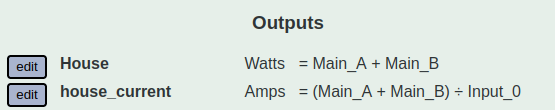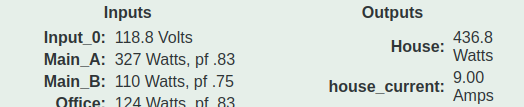# Trying to find total current into house as an output, can't get math to work - explained

Typical US split phase power here.

I have an output set up to sum my two main phases, and another to take that sum and divide it by voltage to get total current into the house:However, the result doesn’t seem to line up with the math:327 + 110 = 437, which matches the “House” output, but then 437 / 118.8 = 3.68, as opposed to the 9 shown.

I’m aware it makes more sense to do two separate calculations for each leg’s current, but this was my starting point, and any output I create that divides by Input_0 seems to off in the same way.

IoTaWatt measures and saves Amps in addition to several other metrics like VA, VAR, Wh and of course Watts. When you define an output, you specify the units of the result which is also the units of any values retrieved for any inputs specified in the script.

In this case, if you simply define the output as:

`House_current. Amps = Main_A + Main_B`

You will get the total of the Amps drawn through the two mains. But that won’t equal Watts / Volts. That’s because Amps * Volts = VA. If you create an output

`House_VA VA = Main_A + Main_B`

You should get a value that will agree somewhat with Amps when divided by volts manually. You cannot divide any of these metrics by Volts within a script because the script will try to return the value of Input_0 in the units specified for the script, which is undefined.

It’s complicated.

Ahhh ok thanks, that makes a lot of sense, and also answers how I can access those other units I could see in the drop down like VA and Hz.

So now if I define an output for Amps of Phase B, and then multiple that by volts (manually), that’s my apparent power, which I can multiply by PF to get the real power, which is what the inputs display on the status page. Everything agrees, and makes sense now, thanks.